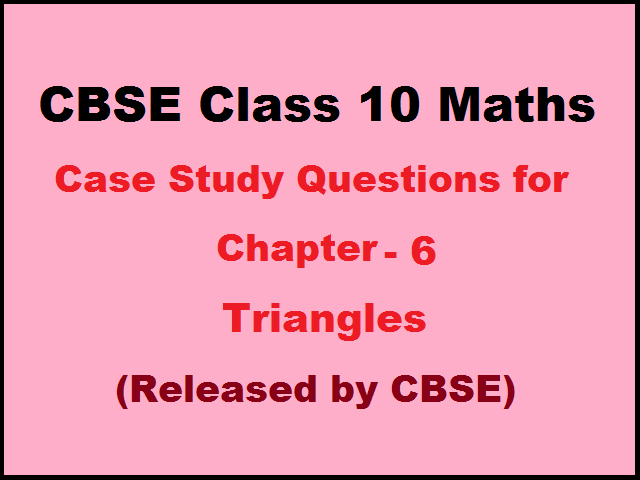# CBSE Class 10 Maths Case Study Questions for Maths Chapter 6 (Published by CBSE)

0
9693CBSE Class 10 Maths Case Study Questions for Chapter 6 – Triangles are available here. Students must practice with these questions to perform well in their Maths exam. All these case study questions have been published by the Central Board of Secondary Education (CBSE). For the convenience of students, all the questions are provided with answers. Also Check Chhattisgarh Pre Pharmacy Test .

Case Study Questions for Class 10 Maths Chapter 6 – Triangles

CASE STUDY 1:

Vijay is trying to find the average height of a tower near his house. He is using the properties of similar triangles. The height of Vijay’s house if 20m when Vijay’s house casts a shadow 10m long on the ground. At the same time, the tower casts a shadow 50m long on the ground and the house of Ajay casts 20m shadow on the ground. Also check Master in Rural Development .

1. What is the height of the tower?

a) 20m

b) 50m

c) 100m

d) 200m

2. What will be the length of the shadow of the tower when Vijay’s house casts a shadow of 12m?

a) 75m

b) 50m

c) 45m

d) 60m

3. What is the height of Ajay’s house?

a) 30m

b) 40m

c) 50m

d) 20m

4. When the tower casts a shadow of 40m, same time what will be the length of the shadow of Ajay’s house?

a) 16m

b) 32m

c) 20m

d) 8m

5. When the tower casts a shadow of 40m, same time what will be the length of the shadow of Vijay’s house?

a) 15m

b) 32m

c) 16m

d) 8m

CASE STUDY 2:

Rohan wants to measure the distance of a pond during the visit to his native. He marks points A and B on the opposite edges of a pond as shown in the figure below. To find the distance between the points, he makes a right-angled triangle using rope connecting B with another point C are a distance of 12m, connecting C to point D at a distance of 40m from point C and the connecting D to the point A which is are a distance of 30m from D such the ADC=900.

Also Check Human Resource Management College in Kolkata .

1. Which property of geometry will be used to find the distance AC?

a) Similarity of triangles

b) Thales Theorem

c) Pythagoras Theorem

d) Area of similar triangles

2. What is the distance AC?

a) 50m

b) 12m

c) 100m

d) 70m

3. Which is the following does not form a Pythagoras triplet?

a) (7, 24, 25)

b) (15, 8, 17)

c) (5, 12, 13)

d) (21, 20, 28)

4. Find the length AB?

a) 12m

b) 38m

c) 50m

d) 100m

5. Find the length of the rope used.

a) 120m

b) 70m

c) 82m

d) 22m

SCALE FACTOR

Case study:

A scale drawing of an object is the same shape at the object but a different size. The scale of a drawing is a comparison of the length used on a drawing to the length it represents. The scale is written as a ratio. The ratio of two corresponding sides in similar figures is called the scale factor

Scale factor= length in image / corresponding length in object

If one shape can become another using revising, then the shapes are similar. Hence, two shapes are similar when one can become the other after a resize, flip, slide or turn. In the photograph below showing the side view of a train engine. Scale factor is 1:200

This means that a length of 1 cm on the photograph above corresponds to a length of 200cm or 2 m, of the actual engine. The scale can also be written as the ratio of two lengths. Also Check Turner Trade .

1. If the length of the model is 11cm, then the overall length of the engine in the photograph above, including the couplings (mechanism used to connect) is:

a) 22cm

b) 220cm

c) 220m

d) 22m

2. What will affect the similarity of any two polygons?

a) They are flipped horizontally

b) They are dilated by a scale factor

c) They are translated down

d) They are not the mirror image of one another.

Answer: d)They are not the mirror image of one another

3. What is the actual width of the door if the width of the door in photograph is 0.35cm?

a) 0.7m

b) 0.7cm

c) 0.07cm

d) 0.07m

4. If two similar triangles have a scale factor 5:3 which statement regarding the two triangles is true?

a) The ratio of their perimeters is 15:1

b) Their altitudes have a ratio 25:15

c) Their medians have a ratio 10:4

d) Their angle bisectors have a ratio 11:5

Answer: b)Their altitudes have a ratio 25:15

5. The length of AB in the given figure:

a) 8cm

b) 6cm

c) 4cm

d) 10cm